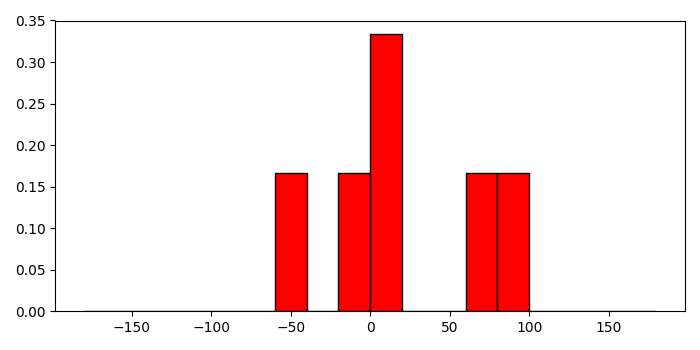# Getting information for bins in Matplotlib histogram function

MatplotlibPythonData Visualization

#### Python Data Science basics with Numpy, Pandas and Matplotlib

Most Popular

63 Lectures 6 hours

#### Data Visualization using MatPlotLib & Seaborn

11 Lectures 4 hours

#### MatPlotLib with Python

9 Lectures 2.5 hours

To get information for bins in matplotlib histogram function, we can take the following steps −

• Create a list of numbers for data and bins.

• Compute the histogram of a set of data using histogram() method.

• Get the hist and edges from the histogram (step 2).

• Find the frequency in a histogram.

• Make a bar with bins (Step 1) and freq (step 4) data.

• To display the figure, use show() method.

## Example

import numpy as np
from matplotlib import pyplot as plt
plt.rcParams["figure.figsize"] = [7.00, 3.50]
plt.rcParams["figure.autolayout"] = True
a = [-0.125, .15, 8.75, 72.5, -44.245, 88.45]
bins = np.arange(-180, 181, 20)
hist, edges = np.histogram(a, bins)
freq = hist/float(hist.sum())
plt.bar(bins[:-1], freq, width=20, align="edge", ec="k", color='red')
plt.show()

## Output